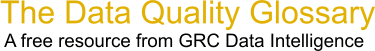Choose Index below for a list of all words and phrases defined in this glossary.

# Z Score

index | Index

## Z Score - definitions

Z Score - A measure of the distance in standard deviations of a sample from the mean. Calculated as (X - X bar) / sigma.

[Category=Data Quality ]

Source: iSixSigma, 06 March 2011 09:54:27, http://www.isixsigma.com/index.php?option=com_glossaryThese advertisers support this free service

z-score - [statistics] A statistical measure of the spread of values from their mean, expressed in standard deviation units, where the z-score of the mean value is zero and the standard deviation is one. In a normal distribution, 68 percent of the values have a z-score of plus or minus 1, meaning they lie within one standard deviation of the mean. Ninety-five percent of the values have a z-score of plus or minus 1.96, meaning they lie within two standard deviations of the mean; 99 percent of the values have a z-score of plus or minus 2.58. Z-scores are a common scale on which different distributions, with different means and standard deviations, can be compared.

[Category=Geospatial ]

Source: esri, 16 October 2012 09:30:14, http://support.esri.com/en/knowledgebase/GISDictionary/term/abbreviationData Quality Glossary.  A free resource from GRC Data Intelligence. For comments, questions or feedback: dqglossary@grcdi.nl